# Truncated Archimedeans

In a previous post,we looked at the Platonics as portions were cut off (truncated). There are four objects that are made from further removal of edges/corners.

Unfortunately, these are not “perfect” truncations.

If you take a cuboctahedron and cut off each corner at the midpoint of each edge, you then end up with smaller triangle faces ¼th the original area and inverted and smaller squares ½ the original area and rotated 45°. You also get 12 new faces that are rectangles. This does not fit the definition of a “regular polyhedron” as rectangles are not regular polygons, they have sides that are of different lengths.

In order to get this object to be regular, you have to distort it slightly to make the rectangles into squares. Then you have a small rhombicuboctahedron. It is called this because the faces lie in the same plane as the rhombic dodecahedron, the dual of the cuboctahedron. (No one ever said that mathematicians were good at naming things.)

A better method of construction is a sort of “double truncation.” take a cube and chamfer all the edges at 45° where the cut is $$(edge length\cdot [\sqrt2-1] )$$ from each edge (Fig.1). You then truncate a second time cutting off each end of the elongated hexagons, meeting each square at the very tip of the corners, creating equilateral triangles (Fig.2).

Looking at any square face straight on, the object has the outline of a regular octagon, therefore the dihedral angle between any two squares is 135°.

Likewise, you can cut off the corners of a cuboctahedron to turn the triangle faces into hexagons and square faces into octagons, but again the new faces are rectangles, not squares. Distortion will then give you a great rhombicuboctahedron. It is also called by some a truncated cuboctahedron.

The octagon-square dihedral angle is 135°.

The white faces of both are parallel to those of an octahedron, so both can also be made from chamfering an octahedron’s edges and truncating the corners.

Another two objects can be made starting with an icosidodecahedron, instead of a cuboctahedron, the small and great rhombicosidodecahedrons and truncating appropriately.

Regardless of how you construct the objects, all faces should be regular polygons and all edges are the same length.

# Nested roots, part 2

I have recently came across a problem involving cube roots of numbers added to square roots, in the form of $$\sqrt{X+Y\sqrt{Z}}$$, this is called a “nested radical.” I already figured out a nested square root, I decided to give this a go.

I can take a number like $$(1+\sqrt5)^2$$ and work out it is equal to $$16 + 8\sqrt5$$. Unfortunately, it is very difficult to recognize that $$1+\sqrt5$$ is the cube root (or even a factor) of that. Even harder, trying to find an equation such as $$\sqrt{1080-624\sqrt3}$$.

Recently, I developed a method of breaking down the equation into its cube root equation.

With an equation in the form of $$\sqrt{X+Y\sqrt{Z}}$$, where Z contains no perfect square factors.

\begin{align} \sqrt{X+Y\sqrt{Z}} &= A+B\sqrt{Z} \\ X+Y\sqrt{Z} &= (A+B\sqrt{Z})^3 \\ &=A^3 + 3A^2B\sqrt{Z} + 3AB^2Z + B^3Z\sqrt{Z} \\ &=A^3 + 3AB^2Z + 3A^2B\sqrt{Z} + B^3Z\sqrt{Z} \\ &=\color{Yellow}{A^3 + 3AB^2Z} +\color{cyan}{( 3A^2B + B^3Z})\sqrt{Z} .\\ \end{align}

We need to find
\begin{align} X &= \color{Yellow}{A^3 + 3AB^2Z} \tag{1}\label{1} \\ Y &=\color{cyan}{3A^2B + B^3Z}. \tag{2}\label{2}\\ \end{align}

If You look at X, You see it is the sum of a cube and a multiple of 3Z.

Let’s look at an example, $$\sqrt{1080-624\sqrt{3}}$$. If we subtract cubes from the initial number(1080) we get:

\begin{align} 1080-1^3 &= 1080-1= 1079 &\color{red}{No} \\ 1080-2^3 &= 1080-8= 1072 &\color{red}{No}\\ 1080-3^3 &= 1080-27=1053=3Z\cdot 117 &\color{lime}{Yes} \\ 1080-4^3 &= 1080-64=1016 &\color{red}{No}\\ 1080-5^3 &= 1080-125=955 &\color{red}{No}\\ 1080-6^3 &= 1080-216=864=3Z\cdot 96 &\color{lime}{Yes} \\ 1080-7^3 &= 1080-343=737 &\color{red}{No}\\ 1080-8^3 &= 1080-512=568 &\color{red}{No}\\ 1080-9^3 &= 1080-729=351=3Z\cdot 39 &\color{lime}{Yes} \\ 1080-10^3 &= 1080-1000=80 &\color{red}{No}\\ 1080-11^3 &= 1080-1331=-251 &\color{red}{No}\\ \end{align}

We need a result that is evenly divisible by 3Z, or 9 in this case. $$11^3$$ is greater than 1080, so we stop. (Even if all answers are complex, will still be Real.)

Our results show 3, 6, and 9 are viable solutions for a, we must now check each against X to get B.

 if(A=3) $$1053 =9\cdot 3\cdot B^2$$ B²=39 $$B=\sqrt{39}$$ if(A=6) $$864 =9\cdot 6\cdot B^2$$ B²=16 $$B=4$$ if(A=9) $$351 =9\cdot 9\cdot B^2$$ $$B^2=4\frac13$$ $$B=\sqrt{\frac{13}{3}}$$

The only even square root is for A=6, B=4. $$X= 6^3 + 3\cdot6 \cdot 4^2 \cdot 3 =1080$$, so this is a possible solution pair. First we must now check against Y.

\begin{align} Y &=3A^2B + B^3Z\\ &=3\cdot 6^2\cdot4 + 4^3\cdot3\\ &=3\cdot 36\cdot4 + 64\cdot3\\ &=432 + 192\\ &=624\\ \end{align}

It matches, so we have a correct solution for $$\sqrt{1080-624\sqrt{3}} =6-4\sqrt{3}$$. Remember, as the original had a negative Y, the solution must negate B.

If our example $$\sqrt{1080-624\sqrt{3}}$$ was actually $$\sqrt{1080-623\sqrt{3}}$$, then the formulas above would not work (there would not be any solution) and would not be able to be simplified any further.

Let’s try $$\sqrt{\frac{371}{125}+\frac{319}{100}\sqrt2 }$$, which can also be written $$\sqrt{2.968+3.19\sqrt2 }$$. This is a bit harder as we are not dealing with integers. It may be easier to try smaller cubes.

\begin{align} 2.968-.1^3 &= 2.968-.001= 2.967=3Z\cdot 0.4945 \\ 2.968-.2^3 &= 2.968-.008= 2.960\\ 2.968-.3^3 &= 2.968-.027= 2.941\\ 2.968-.4^3 &= 2.968-.064= 2.904=3Z\cdot 0.4840\\ 2.968-.5^3 &= 2.968-.125= 2.843\\ 2.968-.6^3 &= 2.968-.216= 2.752\\ 2.968-.7^3 &= 2.968-.343= 2.625=3Z\cdot 0.4375\\ 2.968-.8^3 &= 2.968-.512= 2.456\\ 2.968-.9^3 &= 2.968-.729= 2.239\\ 2.968-1.0^3 &= 2.968-1.000= 1.968=3Z\cdot 0.328\\ 2.968-1.1^3 &= 2.968-1.331= 1.637\\ 2.968-1.2^3 &= 2.968-1.728= 1.240\\ 2.968-1.3^3 &= 2.968-2.197= 0.771=3Z\cdot 0.1285\\ 2.968-1.4^3 &= 2.968-2.744= 0.224\\ 2.968-1.5^3 &= 2.968-3.375=-0.407 \\ \end{align}

Of these, 0.1, 0.4, 0.7, 1, and 1.3 are finitely divisible by 6 (3Z). If we didn’t find any, we would have to try smaller numbers.

 if (A=0.1) $$2.967=6\cdot 0.1\cdot B^2$$ $$B^2=\frac{4945}{1000}$$ B is irrational if (A=0.4) $$2.904=6\cdot 0.4\cdot B^2$$ $$B^2=\frac{121}{100}$$ $$B=\frac{11}{10}$$ if (A=0.7) $$2.625=6\cdot 0.7\cdot B^2$$ $$B^2=\frac58$$ $$B=\sqrt{\frac58}$$ if (A=1) $$1.968=6\cdot 1\cdot B^2$$ $$B^2=\frac{41}{125}$$ B is irrational if (A=1.3) $$0.771=6\cdot 1.3\cdot B^2$$ $$B^2=\frac{257}{2600}$$ B is irrational

Three of these are obviously irrational, so cannot be part of our solution, so are not calculated.

For A=0.7, B is also irrational, so we will first test A=0.4:

\begin{align} 2.968 &= A^3 + 3AB^2Z\\ &=0.4^3 +3\cdot 0.4\cdot B^2\cdot 2\\ &=0.064 + 2.4\cdot B^2\\ 2.904&=2.4B^2\\ 1.21&=B^2\\ B&=\frac{11}{10}=1.1\\ \end{align}

And for Y:

\begin{align} Y &=3A^2B + B^3Z\\ &=3\cdot 0.4^2\cdot1.1 + 1.1^3\cdot2\\ &=3\cdot 0.16\cdot1.1 + 1.331\cdot2\\ &=0.528 + 2.662\\ &=3.19\\ \end{align}

It matches, so we have a correct solution for $$\sqrt{2.968+3.19\sqrt2 } =0.4+1.1\sqrt{2}$$.

Any cube root in this form must also have $$X^2+Y^2\cdot Z$$ equal a perfect cube.

$$\sqrt{1080^2-624^2\cdot 3}= -12$$ and $$\sqrt{\frac{371}{125}^2-\frac{319}{100}^2\cdot2}=\frac{-113}{50}$$, so these are solvable.

Also, remember that these are cube roots, so there are two complex roots. Just rotate the answer found by 120° and 240°, for the remaining answers. If this technique doesn’t find a simple answer, then all 3 may be complex.

Another way to solve, if Z=5 and the number under the radical is in the form of $$2K\pm K\sqrt5$$, aka $$K\cdot(2\pm \sqrt5)$$, the answer will be in the form of $$A\pm +A\sqrt5$$. In this case, $$A=\sqrt{\frac{K}{8}}$$.

Alternate values for Z:

 Z Form A= 2 $$K(7\pm 5\sqrt2)$$ $$\sqrt{K}$$ 3 $$K(5\pm 3\sqrt3)$$ $$\sqrt{\frac{K}{2}}$$ 5 $$K(2\pm \sqrt5)$$ $$\sqrt{\frac{K}{8}}$$ 6 $$K(19\pm 9\sqrt6)$$ $$\sqrt{K}$$ 7 $$K(11\pm 5\sqrt7)$$ $$\sqrt{\frac{K}{2}}$$ 8 $$K(25\pm 11\sqrt8)$$ or $$K(25\pm 22\sqrt2)$$ $$\sqrt{K}$$ 10 $$K(31\pm 13\sqrt{10})$$ $$\sqrt{K}$$

This is likely not the best method for finding the cube roots, but it does work.

# Prisms and Antiprisms

In geometry, a prism is a polyhedron with an n-sided polygonal base, another congruent parallel base (with the same rotational orientation), and n other faces (necessarily all parallelograms) joining corresponding sides of the two bases. Prisms are named for their base, so a prism with a pentagonal base is called a pentagonal prism.

In regular prisms, all side faces (all squares) are at right angles to the bases. The number of prisms is infinite, approaching a circular base.

The interior angles of the base faces is $$\frac{180(n-2)}{n}$$, where n is the number of sides. This is really the only “difficult” math property of prisms.

Some prisms are not normally considered or called prisms, such as a square prism is just a cube.

If instead of connecting the bases with squares, you connect them with equilateral triangles, you then get antiprisms.

For n=2, the digonal antiprism is a degenerate case, as there is no base polygon, only a line segment. Otherwise the top face is a duplicate of the bottom, only rotated 180°/n.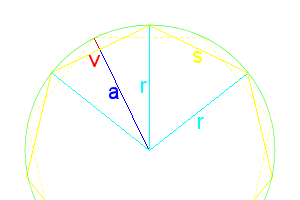Figure 1: Polygon Dimensions

For dihedral angles for antiprisms, things are a lot more complicated than prisms.

A regular polygon always has the following properties:

\begin{align} radius\ r&=DB=\frac{s}{2\sin\frac{180^\circ}{n}} = \frac{a}{\cos\frac{180^\circ}{n}} \\ apothem\ a &=\overline{DE}=r\cdot\cos\frac{180^\circ}{n}=\frac{s}{2\tan\frac{180^\circ}{n}} \\ side\ s&=r\cdot2\sin\frac{180^\circ}{n}=a\cdot 2\tan\frac{180^\circ}{n}=AB=BC=FG \\ angle\ \theta &=\frac{360^\circ}{n} \\sagitta\ v&=r-a=r(1-\cos\frac{360}{2n})=r(2\sin^2\frac{360}{4n})=\frac{s(1-\cos\frac{360}{2n})}{2\sin\frac{360}{2n}}=\frac{s(\sin^2\frac{360}{4n})}{\sin\frac{360}{2n}}=s\frac12\tan\frac{90}{n}=\overline{BE} \end{align}

The apothem is the distance from the center to the midpoint of any side. The difference between the radius and apothem is called the sagitta, also sometimes called the versine function ($$versin\ \mu=1-\cos\mu=2\sin^2\frac12\mu)$$. The antiprism’s sides will be deflected away from 90° by the length of BE (or v). The dotted line is the location of the second base, always rotated θ/2 to that of the first base.

Normally it would be odd trying to calculate the radius of a circle, but we are defining our polygon based on its side length being one unit.

If we draw a line at the midpoint of each triangle all the way around, we get a regular polygon with 2n sides, parallel to each base.

The height of the triangle is line BE2, $$\frac{\sqrt3}{2}$$. We do not yet know the height of the antiprism ($$\overline{E_1E_2}$$), but can find it.

\begin{align} (\overline{BE_1})^2+(\overline{E_1 E_2})^2 &=(\overline{BE_2})^2 \\ (\overline{E_1 E_2})^2 &=(\overline{BE_2})^2-(\overline{BE_1})^2 \\ &=\left(\frac{\sqrt3}{2}\right)^2-\left(s\frac12\tan\frac{90}{n}\right)^2 \\ &=\frac34-s^2\cdot\frac14\tan^2\frac{90}{n} \\ &=\frac{3-s^2\tan^2\frac{90}{n}}{4} \\ \overline{E_1 E_2} &=\frac12\sqrt{3-s^2\tan^2\frac{90}{n} } \end{align}

This is as simplified as we can get without deciding on how many sides the base will have. The height of an antiprism must always be less than $$\frac12 s\sqrt3$$, the height of a regular triangle.

Here are a few of the first heights for n:

 n θ Height 2 45 $$\frac1{\sqrt2}$$ 3 60 $$\sqrt{\frac23}$$ 4 90 $$\sqrt{\frac12}$$ 5 36 $$\sqrt{\frac{5+\sqrt5}{10}}$$ 6 30 $$\sqrt{\sqrt3-1}$$ 8 22.5 $$\sqrt{\sqrt{5+\frac{7\sqrt2}{2}} -1-\sqrt2}$$ 10 18 $$\frac12\sqrt{-8-4\sqrt5-2(1+\sqrt5)\sqrt{5+2\sqrt5}}$$

For the dihedral angles, ∠E1BE2 is the same as ∠Ψ, and $$\cos\angle BE_2D_2 =-\cos\angle\Psi$$.

\begin{align} \cos\angle E_1BE_2 &=\frac{(BE_1)^2+(BE_2)^2-(E_1 E_2)^2}{2[(BE_1)(BE_2)]} \\ &=\frac{\left(\frac12\tan\frac{90}{n}\right)^2+\left(\frac{\sqrt3}{2}\right)^2-\left(\frac12\sqrt{3-\tan^2\frac{90}{n} }\right)^2}{2\cdot\frac12\tan\frac{90}{n}\cdot\frac{\sqrt3}{2}} \\ &=\frac{ \frac{\tan^2\frac{90}{n}}{4}+\frac34-\frac{3-\tan^2\frac{90}{n} }{4} }{\frac{\sqrt3}{2}\cdot\tan\frac{90}{n}} \\ &=\frac{ \frac{2\tan^2\frac{90}{n}}{4} }{\frac{\sqrt3}{2}\cdot\tan\frac{90}{n}}=\frac{ \frac12\tan^2\frac{90}{n} }{\frac{\sqrt3}{2}\cdot\tan\frac{90}{n}} \\ &= \frac{\tan^2\frac{90}{n}}{2} \cdot \frac{2}{\sqrt3\cdot\tan\frac{90}{n}} \\ &= \frac{\tan^2\frac{90}{n}}{\sqrt3\cdot\tan\frac{90}{n}} \\ &= \frac{\tan\frac{90}{n}}{\sqrt3} \\ &= \frac1{\sqrt3}\cdot\tan\frac{90}{n} \\ \cos\angle BE_2D_2&=-\frac1{\sqrt3}\cdot\tan\frac{90}{n} \end{align}

Here are the first few dihedrals for n:

 n $$\angle E_1BE_2$$ $$\angle BE_2D_2$$ 2 54.735° 125.264° 3 70.528° 109.471° 4 76.163° 103.836° 5 79.187° 100.812° 6 81.101° 98.8994° 8 83.405° 96.5945°

For the side-to-side dihedrals, let’s draw a line from point C, straight down, equal to the height, and call this point K. K is in the same plane as the bottom base. Looking at Figure 1, we can see that ∠KDF is $$\frac32\theta$$, or $$\frac{540}{n}$$. Connecting F and K, we get a line of $$2r\sin\frac{540}{2n}= s\cdot\frac{sin\frac{270}{n}}{\sin\frac{180}{n}}$$. Note $$\overline{FK}$$ is coplanar with G and E2, but does not go through either point.

We can now find the distance between C and F,

\begin{align} (CF)^2&=(CK)^2+(FK)^2 \\ &=\frac{3-s^2\tan^2\frac{90}{n}}{4} + s^2\cdot\frac{\sin^2\frac{270}{n}}{\sin^2\frac{180}{n}} \\ (CF) &=\sqrt{\frac{3-s^2\tan^2\frac{90}{n}}{4} + s^2\cdot\frac{\sin^2\frac{270}{n}}{\sin^2\frac{180}{n}} } \end{align}

In Figure 2, we see that $$CL=LF=BE_2=\frac{\sqrt3}{2}$$. We can now find ∠CLF, the dihedral, with s=1 factored out,

\begin{align} \cos\angle CLF &=\frac{(CL)^2+(LF)^2- (CF)^2}{2\cdot CL\cdot LF} \\ &=\frac{(\frac{\sqrt3}{2})^2+(\frac{\sqrt3}{2})^2-(CF)^2 }{2\cdot (\frac{\sqrt3}{2})(\frac{\sqrt3}{2})} \\ &=\frac{\frac34+\frac34-(CF)^2 }{\frac32} \\ &=\left[\frac64-(CF)^2\right]\div\frac32=\left[\frac32-(CF)^2\right]\cdot\frac23 \\ &=1-\frac23(CF)^2 \\ &=1-\frac23\left(\frac{3-\tan^2\frac{90}{n}}{4} + \frac{\sin^2\frac{270}{n}}{\sin^2\frac{180}{n}} \right) \end{align}

Multiplying out the CF2 is really messy, best to reduce the other parts as much as possible, then square.

Here are the first few dihedral angles for n:

 n $$\cos\angle CLF$$ ∠CLF 2 $$\frac13$$ 70.528779 3 $$-\frac13$$ 109.47122 4 $$\frac{1-2\sqrt2}{3}$$ 127.552 5 $$-\frac{\sqrt5}{3}$$ 138.189685

# Truncated Platonics

There are several Archimedean solids that are formed by the truncation (cutting off) of each corner of a Platonic solid.

These can be shown in successive truncations from one shape to its dual.

Initial truncations remove a pyramid from each corner the original object, the base of which is a regular polygon. If the original face is a triangle, the resultant edges will be $$\frac13$$ the original edge; if square, $$\sqrt\frac12$$; and if a pentagon, $$\sqrt\frac15$$.

Note: Each truncation makes the object smaller, but for the math below, the presumption is an object with edge length of 1.

Complete truncation (also called “rectified”) completely removes original edges, new faces meet a the midpoint of original edges.

Note that the left objects are “sitting” on a face, and the right objects are “standing” on a vertex. This is the result of matching the vertices of one to the midpoints of the dual.

In quasi-regular polyhedra, truncation is not necessarily resultant in regular faces. Adjustments are made to make the faces become regular, these are called rhombi-truncations.

The dihedral angles of the “main” faces (red/red or yellow/yellow) of the truncated object are the same as the original object’s dihedral angles. If there are only three faces at a vertex, the vertex angles are easily found with the formula: $$\Large{\cos\theta=\frac{\cos\epsilon}{\cos(\frac{\mu}{2})}}$$, where θ is the vertex angle of the newly created face to the opposing edge, ε is the interior angle of the new main face, and μ is the interior angle of the newly created face.

The dihedral angles of the new faces are a bit harder. We have to do each separately.

Since each truncation removes a pyramid with a regular base, we can calculate the dihedral angle of one side to the base, giving us the complimentary angle to that of the dihedral angle of the new/main faces of the polyhedra. Remember that if A+B=180, then cos A = —cos B, so we can find the cosine of the pyramid’s dihedral angle, then negate it.

I’ve already done the math for the tetrahedron, the cosine of the dihedral is $$\frac13$$, or $$70.528779\ldots^\circ$$, so the dihedral cosine for the hexagon-triangle faces of the truncated tetrahedron would be $$-\frac13$$, or $$109.47122\ldots^\circ$$. The two do add up to 180° and the latter is the same as the rectified tetrahedron (aka octahedron), therefore we are on the right track.

For the trunc. cube, a triangular based pyramid with right angles at the apex, would have a base dihedral of $$cos^{-1}(\frac{1}{\sqrt3})$$, so the octagon-triangle dihedral would be $$cos^{-1}(\frac{-1}{\sqrt3})$$, or 125.2643897…°.

The trunc. octahedron has a square pyramid (Johnson solid J1) removed that equates to a half octahedron. The dihedral for J1 is $$\cos^{-1}(\frac{1}{\sqrt3})$$, so the trunc. octahedron hex-square dihedral would be $$\cos^{-1}(\frac{-1}{\sqrt3})$$, or 125.2643897…°.

Yes, these are the same two angles, which can be seen in the cuboctahedron, all edges are square-triangles, so must be the same. It is also evident when you realize that corresponding faces are parallel from one shape to the next during truncation.

For a trunc. dodecahedron, the decagon-decagon faces have the same dihedral as the dodecahedron. The decagon-triangle edges have the same dihedral as the pent-hex edges of the trunc. icosahedron, 142.622632°, but we will check this later below.

The vertex angle θ is found by

\begin{align}\cos\theta &=\frac{\cos108}{\cos\frac{108}{2}}=\frac{-\cos72}{\cos54} \\&=-\frac{\frac{\sqrt5-1}{4}}{\sqrt{\frac{5-\sqrt5}{8}}} \\&=-\frac{\sqrt5-1}{4}\cdot\sqrt{\frac{8}{5-\sqrt5}} \\&=-\frac{\sqrt8 \cdot (\sqrt5-1)}{4\sqrt{5-\sqrt5}} \\&=-\frac{\sqrt8 \cdot \sqrt{(\sqrt5-1)^2}}{4\sqrt{5-\sqrt5}} \\&=-\frac{\sqrt8 \cdot \sqrt{6-2\sqrt5} }{4\sqrt{5-\sqrt5}} \\&=-\frac{\sqrt8 \cdot \sqrt2\cdot\sqrt{3-\sqrt5} }{4\sqrt{5-\sqrt5}} \\&=-\frac{\sqrt{16} \cdot\sqrt{3-\sqrt5} }{4\sqrt{5-\sqrt5}} \\&=-\frac{4 \cdot\sqrt{3-\sqrt5} }{4\sqrt{5-\sqrt5}} \\&=-\frac{\sqrt{3-\sqrt5} }{\sqrt{5-\sqrt5}} \\&=-\sqrt{\frac{3-\sqrt5 }{5-\sqrt5} } \\&=-\sqrt{\frac{3-\sqrt5 }{5-\sqrt5} \cdot \frac{5+\sqrt5}{5+\sqrt5} } \\&=-\sqrt{\frac{10-2\sqrt5 }{20} } \\&=-\sqrt{\frac{5-\sqrt5 }{10} } \\ \end{align}

So the vertex angle is 121.7174744…°

The trunc. icosahedron we already did the work for, the vertex angles are 148.2825255…°.

We already know the dihedrals of the cuboctahedron and icosidodecahedron are the same as their truncated counterparts, but what if we wanted to verify that?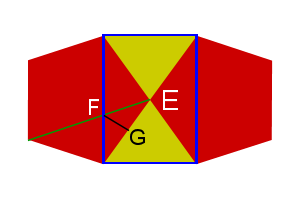Figure 1: Icosidodecahedron vertex

As Fig. 1 shows, we can draw a rectangle onto the surface of a icosidodecahedron, with points ABCD, which creates a pyramid with apex E. As lines AD and BC cross the width of the pentagons, their length is then $$Phi\ (\phi)\ or\ \frac{1+\sqrt5}{2}$$. The green line is also Φ and crosses AD at point F. Point G is the midpoint of line DE, making DG=EG=1/2.

Notice that the blue and green lines are parallel to the upper and left sides of the pentagon, making a parallelogram, making AF=1, so $$DF=\phi-1 = \frac{\sqrt5-1}{2}$$. ∠DGF is 90°, we can find FG,

\begin{align}DG^2+FG^2 &=DF^2 \\FG^2&=DF^2-DG^2 \\&=\left(\frac{\sqrt5-1}{2}\right)^2-\frac1{2^2} \\&=\frac{6-2\sqrt5}{4} – \frac14 \\&=\frac{5-2\sqrt5}{4} \\FG&=\frac{\sqrt{5-2\sqrt5}} {2} \end{align}

The distance between F and C is

\begin{align}CF^2&=CD^2+DF^2 \\&=1+(\frac{\sqrt5-1}{2})^2 \\&=\frac44 + \frac{6-2\sqrt5}{4} \\&=\frac{10-2\sqrt5}{4} \\CF&=\frac{\sqrt{10-2\sqrt5}} {2} \end{align}

Line CG is the same as the height of the triangle, $$\frac{\sqrt3}{2}$$. Now we can find the dihedral (∠FGC)

\begin{align}\cos\angle FGC&=\frac{FG^2+CG^2-CF^2}{2\cdot FG\cdot CG} \\&=\frac{ \frac{5-2\sqrt5}{4}+\frac34-\frac{10-2\sqrt5}{4}} {2\cdot\frac{\sqrt{5-2\sqrt5}} {2}\cdot\frac{\sqrt3}{2}} \\&=\frac{ \frac{5-2\sqrt5+3-10+2\sqrt5}{4}} {(\sqrt{5-2\sqrt5})\cdot\frac{\sqrt3}{2}} \\&=\frac{-\frac24}{\sqrt{5-2\sqrt5}\cdot\frac{\sqrt3}{2}} = -\frac24\div \left(\frac{\sqrt3\sqrt{5-2\sqrt5}} {2}\right) \\&=-\frac12 \cdot \left(\frac{2}{\sqrt3\sqrt{5-2\sqrt5}}\right) \\&=\frac{-1}{\sqrt3 \sqrt{5-2\sqrt5}} \\&=\frac{-1}{\sqrt3 \sqrt{5-2\sqrt5}}\cdot \frac{\sqrt{5+2\sqrt5}}{\sqrt{5+2\sqrt5}} \\&=\frac{-\sqrt{5+2\sqrt5}}{\sqrt3 \sqrt{25-4\sqrt5-20-4\sqrt5}} \\&=\frac{-\sqrt{5+2\sqrt5}}{\sqrt3 \sqrt5} \\&=-\sqrt{\frac{5+2\sqrt5}{15} } \\\angle FGC&=142.62263\ldots^\circ \end{align}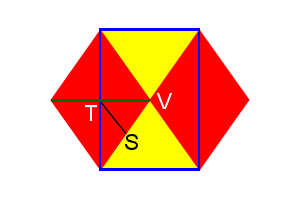Figure 2: Cuboctahedron vertex

Just like the icosidodecahedron, we can draw a rectangle onto the surface of a cuboctahedron (Fig. 2), with points WXYZ, which creates a pyramid with apex V. As lines WZ and XY cross the diagonals of the squares, their length is then $$\sqrt2$$. The green line is also $$\sqrt2$$ and crosses WZ at point T. Point S is the midpoint of line WV, making WS=VS=1/2.

Line ST is half the width of the square, so its length is ½. Lines TV and TW are $$\frac{\sqrt2}{2}\ or \frac1{\sqrt2}$$.

The distance between T and X is

\begin{align}TX^2&=WX^2+TW^2 \\&=1+(\frac1{\sqrt2})^2 \\&=\frac22 + \frac12 \\&=\frac32 \\TX&=\sqrt{\frac32} \end{align}

Line SX is the same as the height of the triangle, $$\frac{\sqrt3}{2}$$. Now we can find the dihedral (∠TSX)

\begin{align}\cos\angle TSX&=\frac{ST^2+SX^2-TX^2}{2\cdot ST\cdot SX}=\frac{ \left(\frac12\right)^2+\left(\frac{\sqrt3}{2}\right)^2-\left(\sqrt\frac32\right)^2} {2\cdot\frac12\cdot\frac{\sqrt3}{2}} \\&=\frac{\frac14+\frac34-\frac64} {\frac{\sqrt3}{2}} \\&=\frac{-\frac24} {\frac{\sqrt3}{2}} = -\frac24 \cdot \frac2{\sqrt3} \\&=\frac{-1}{\sqrt3} \\\angle TSX&=125.2643897\ldots^\circ \end{align}

# Icosahedron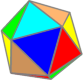The icosahedron has 12 equilateral triangles as faces.

It can be split into 3 parts, a pentagonal anti-prism and two pentagonal pyramids. We will start by looking at a pentagonal pyramid.

We have already done most of the work earlier.

To find ∠ACB,

\begin{align}\cos \angle ACB &= \frac{1^2 + S^2 – H^2}{2\cdot1\cdot S}\\ &= \frac{1^2 + \frac34 – \frac{5+2\sqrt5}{4}}{2\cdot 1\cdot \frac{\sqrt3}{2}}\\ &= \frac{\frac{2-2\sqrt5}{4} }{\sqrt3} \\ &= \frac{2-2\sqrt5}{4\sqrt3} \\ &= \frac{1-\sqrt5}{2\sqrt3}\\ACB &= 110.905157\ldots^\circ \end{align}

This is the vertex angle from one face to the opposite edge.

Taking two triangle faces that are abutting, the tips would be Phi (Φ) apart, you can draw a triangle of sides S, S, and Φ, going through the center of each equilateral triangle and the tips.

\begin{align}Icosahedron\ Dihedral \angle &= \cos^{-1}\Bigg(\frac{S^2 + S^2 – \Phi^2}{2\cdot S\cdot S}\Bigg)\\ &=\cos^{-1}\Bigg(\frac{ \frac34 + \frac34 – \frac{6+2\sqrt5}{4} }{ 2\cdot \frac{\sqrt3}{2} \cdot \frac{\sqrt3}{2} }\Bigg)\\ &=\cos^{-1}\Bigg(\frac{ – \frac{2\sqrt5}{4} }{ 2\cdot \frac34 }\Bigg)\\ &=\cos^{-1}\Bigg(\frac{ – 2\sqrt5 }{ 2\cdot 3 }\Bigg)\\ &=\cos^{-1}\Bigg(\frac{-\sqrt5}{3}\Bigg)\\ &= 138.1896851\ldots^\circ \end{align}

# Dodecahedron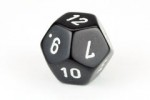The dodecahedron is a regular polyhedron made of 12 regular pentagon faces.

A D12 die is often used in certain role playing games.

Since a dodecahedron has 12 sides, you can print out a calendar, one month per face, and have a conversation piece on your desk. (My advice, use heavy paper and score lines before folding.)

The vertex angles are $$cos^{-1} ( \frac{\cos 108}{\cos \frac{108}{2}} )$$ or 121.7174744…°.

To find the dihedral angles, we will start with a dodecahedron with edge length of 1. Let’s take Figure 1 and slice through the drawn lines going across four of the faces. We get a shape similar to some house roofs, with a square base with sides of length Phi (Φ) or $$\frac{1+\sqrt5}{2}$$, as this is the width of a regular pentagon. There are also two triangles and two trapezoids, each with one edge length of Φ and all others 1. In other words, AE=BE=EF=CF=DF=1 and AB=BD=CD=AC=Φ.

Lengths of the pentagon are in Fig. 3, exact representations are not needed at this time.

If we take triangle ABE and “slide” it up to have it meet triangle CDF at point F, shown in red. We will call the points of the new triangle A’, B’, and F, (those are read as A-prime and B-prime). This gives us a rectangular pyramid A’B’CDF.

We know that line EF is 1, so lines AA’ and BB’ are also 1. Lines AC and BD are Φ, so lines A’C and B’D are length Φ-1 or $$\frac{\sqrt5-1}{2}$$, so B’H and HD are each $$\frac{\sqrt5-1}{4}$$.

Taking the midpoints of A’C and B’D (points G and H, Fig. 5) we can use the Pythagorean theorem to find lengths of these lines,

\begin{align}\left(\frac{\sqrt5-1}{4}\right)^2 + FH^2 &= 1^2 \\ \frac{6-2\sqrt5}{16} + FH^2 &= 1\\ FH^2 &= 1- \frac{6-2\sqrt5}{16} \\ &= \frac{16}{16} – \frac{6-2\sqrt5}{16} \\ &=\frac{10+2\sqrt5}{16} \\ &= \frac{5+\sqrt5}{8} \\ FH &= \sqrt{\frac{5+\sqrt5}{8}} = Cos 18^\circ \end{align}

FG = FH and GH=CD=Φ. This gives us all three sides of triangle GFH, so we use the law of cosines to find ∠GFH,

\begin{align} \cos\angle GFH &= \frac{FG^2 + FH^2 – \Phi^2}{2\cdot FG\cdot FH} \\ &=\frac{\frac{5+\sqrt5}{8} + \frac{5+\sqrt5}{8} – \frac{6+2\sqrt5}{4} }{ 2\cdot \sqrt{\frac{5+\sqrt5}{8}} \cdot \sqrt{\frac{5+\sqrt5}{8}} } \\ &=\frac{\frac{10+2\sqrt5}{8} – \frac{12+4\sqrt5}{8} }{ 2\cdot \frac{5+\sqrt5}{8} } \\ &=\frac{\frac{-2-2\sqrt5}{8} }{ 2\cdot \frac{5+\sqrt5}{8} } \\ &=\frac{-2-2\sqrt5 }{ 2\cdot (5+\sqrt5) } \\ &=\frac{-1-\sqrt5 }{ (5+\sqrt5) } \\ &=\frac{-1-\sqrt5 }{ 5+\sqrt5 }\cdot \frac{-1+\sqrt5}{-1+\sqrt5} \\ &=\frac{1-5+\sqrt5-\sqrt5 }{-5+5-\sqrt5+5\sqrt5} \\ &=\frac{-4}{4\sqrt5}\\ &=\frac{-1}{\sqrt5} \\ \angle GFH &=116.56505\ldots^\circ \end{align}

This is the dodecahedron’s dihedral angle.

# PentagonA regular pentagon has the following dimensions:

AB=BC=CD=DE=AE=BG=EG=1

BF=EF=Φ/2 = cos 36 = sin 54 = $$\frac{1+\sqrt{5}}{4}$$

CG=DG= Φ-1 = 1/Φ = $$\frac{\sqrt5-1}{2}$$

AF=FG= cos 54 = sin 36 = $$\sqrt{\frac{5-\sqrt5}{8}}$$

AG= 2cos 54 = 2sin 36= $$\sqrt{\frac{5-\sqrt5}{2}}$$

CH=DH=BK=CK=1/2

Height AH=EK=$$\frac12\cos 18 = \frac12\sin 72 = \frac12\sqrt{5+2\sqrt5}$$

Circumradius AJ=$$\sqrt{\frac{5+\sqrt5}{10}}$$ = BJ=CJ=DJ=EJ

Inradius JK=HJ= $$\sqrt{\frac{5+2\sqrt5}{20}}$$ = $$\frac{AH}{\sqrt5}$$

FH= cos 18 =sin 72 = $$\sqrt{\frac{5+\sqrt5}{8}}$$

FJ=JK/Φ

GJ=AJ/Φ

GH=AF/Φ=$$\frac12\sqrt{5-2\sqrt5}$$

ABGE is a equilateral rhombus, with angles {108°, 54°, 108°, 54°} and diagonals of length Φ and $$\sqrt{\frac{5-\sqrt5}{2}}$$.

The small pentagon in the center has sides $$1/\Phi^2 = \frac{3-\sqrt5}{2}$$ times that of the larger.

# Factoring numbers with square root terms

Quite often, when doing calculations on polyhedra, you will find yourself with complex equations like $$\frac{20+7\sqrt3}{1+2\sqrt3}$$.

Is the top evenly divisible by the bottom? I certainly can’t tell just by looking at them.

There must be a method to factor the numerator.

Quite often, when doing calculations on polyhedra, you will find yourself with complex equations like $$\begin{equation}\tag{eq1}\label{eq1}\frac{20+7\sqrt3}{1+2\sqrt3}\end{equation}$$

Is the top evenly divisible by the bottom? I certainly can’t tell just by looking at them.

There must be a method to factor the numerator.

Let’s start with $$\color{blue}{(A+B\sqrt{R})}\color{lime}{(C+D\sqrt{R})}$$ multiplying the terms together gives $$\color{salmon}{AC+BRD+(BC+AD)\sqrt{R}}$$

So, $$\frac{\color{salmon}{AC+BRD+(BC+AD)\sqrt{R}}}{\color{blue}{A+B\sqrt{R}}} = \color{lime}{C+D\sqrt{R}}$$

Then we can substitute known values (A=1, B=2, R=3) into the formula. $$\frac{1C+2(3)D+(2C+1D)\sqrt{3}}{1+2\sqrt{3}} = C+D\sqrt{3}$$

Finding the answer is just a matter of finding C and D.

Consider that
$$\frac{\color{yellow}{C+6D} + \color{cyan}{(2C+D)}\sqrt3}{1+2\sqrt3} = \frac{\color{yellow}{20}+\color{cyan}{7}\sqrt3}{1+2\sqrt3}$$

We can break the equation into the colored sections to find \begin{align}\color{yellow}{C+6D} & \color{yellow}{=20} \\ C&=20-6D\tag{eq2}\label{eq2} \end{align} and $$\color{cyan}{2C+D = 7}\tag{eq3}\label{eq3}$$

We now know C \eqref{eq2}, at least in terms of D, then substitute this into \eqref{eq3}.
\begin{align}2(20-6D)+D &= 7 \\ 40-12D+D &= 7 \\ -11D &= 7-40 = -33 \\D &= \frac{-33}{-11} \\ D&=3\end{align}

Returning to \eqref{eq2} and substituting D=3, we get $$C = 20-6\cdot 3 = 20-18 = 2$$.

Then our answer should be $$2+3\sqrt{3}$$, and it is, because $$(1+2\sqrt3)(2+3\sqrt3) = 20+7\sqrt3$$.

This method make it simple to divide out these complicated numbers, although, you might not get a simpler answer. For example, if we change \eqref{eq1} to $$\Large\frac{19+7\sqrt3}{1+2\sqrt3}$$, we end up with answers C=23/11 and D=31/11, but it still works as $$(1+2\sqrt3)(\frac{23}{11}+\frac{31\sqrt3}{11}) = 19+7\sqrt3$$. Although, we could write our answer as $$\frac{1}{11}(23+31\sqrt3)$$.

Another example:$$\frac{-3}{3+2\sqrt3}$$
We have values (A=3, B=2, R=3), giving $$3C + 2\cdot3D + (2C+3D)\sqrt3 = (3+2\sqrt3)(C+D\sqrt3)$$

Remember that $$-3$$ is the same as $$-3+0\sqrt3$$. So
\begin{align}3C+6D&=-3\label{e2}\tag{eq4}\\ 2C+3D&=0\label{e3}\tag{eq5} \end{align}

Working out \eqref{e2} gives
\begin{align}3C+6D&=-3 \\ 6D&= -3-3C \\D&=\frac{3(-1-C)}{6} \\D&= \frac{-1-C}{2}\end{align}

Plugging into \eqref{e3}, gives
\begin{align}2C+3D&=0 \\ 2C+3\cdot(\frac{-1-C}{2})&=0 \\ \frac{4C}{2}+\frac{-3C-3}{2}&=0 \\ \frac{4C-3C-3}{2}&=0 \\ \frac{C-3}{2}&=0 \\ C-3&=0 \\C&=3\end{align}

With C=3, we can find D in \eqref{e2} \begin{align}3(3)+6D&=-3 \\ 9+6D&=-3 \\ 6D&=-3-9 \\ 6D&=-12 \\ D&=\frac{-12}{6} \\ D&=-2\end{align}

So the answer is $$3-2\sqrt3$$, as $$(3+2\sqrt3)(3-2\sqrt3)=-3$$.

You might notice this is the same as the “Difference of 2 squares” (x-y)(x+y) = x²-xy+xy-y² = x²-y². The “xy” terms cancel each other out, leaving 0. (x-y) and (x+y) are conjugates of one another, so when you have a numerator with a zero times the square root (or in other words, the numerator is a whole number), you may wish to try the denominator’s conjugate first. It may not be the answer you seek, but it can help you sometimes.

If the denominator is a whole number (or rational), $$\frac{6+2\sqrt3}{-2}$$

then divide both parts of the numerator by the denominator,

$$\frac{6}{-2}+\frac{2\sqrt3}{-2}=-3-\sqrt3$$

So to find an answer to $$\notag\frac{X+Y\sqrt{R}}{A+B\sqrt{R}} = C+D\sqrt{R}$$

You will need to find the solutions to $$AC+BRD=X$$ and $$BC+AD=Y$$

UPDATE: I have also realized that you can just multiply the top and bottom of the fraction by the denominator’s conjugate.

In the first equation:

\begin{align}\frac{20+7\sqrt3}{1+2\sqrt3}&=\frac{(20+7\sqrt3)(1-2\sqrt3)}{(1+2\sqrt3)(1-2\sqrt3)}\\&=\frac{20-42+7\sqrt3-40\sqrt3}{1-12}\\&=\frac{-22-33\sqrt3}{-11}\\&=2+3\sqrt3\end{align}

In this method, the denominator is always a whole number (if both A&B are whole numbers).

# sqr(A+B*sqr(C))

Lately, I have been doing a lot of math involving square roots of numbers added to square roots, in the form of $$\sqrt{A+B\sqrt{C}}$$, this is called a “nested radical.” Normally, you would not be able to simplify any further, unless there was a common factor of both that could be removed, or if both items had the same number under the radical (eg. $$\sqrt{4\sqrt7+12\sqrt7}=\sqrt{16\sqrt7}=4\sqrt{7}$$ ).

I can take a number like $$(1+\sqrt5)^2$$ and work out it is equal to $$6 + 2\sqrt5$$. Unfortunately, it is very difficult to recognize that $$1+\sqrt5$$ is the square root (or even a factor) of that. Even harder, trying to find an equation such as $$\sqrt{469+144\sqrt5}$$.

Recently, I found a method of breaking down the equation into its square root equation.

With an equation in the form of $$\sqrt{A+B\sqrt{C}}$$, where C contains no perfect square factors, taking the formula $$D^2 = A^2 – (B^2)*C$$, if D is a rational number, you will be able to factor the equation, otherwise it is simplified as much as is possible.

Then you will be able to get the formula: $$\sqrt{A+B\sqrt{C}} = \pm \left(\sqrt{\frac{A-D}{2}} + sign(A*B)*\sqrt{\frac{A+D}{2}}\right)$$, where sign(A*B) is the positive or negative value of A*B, it will always give you 0, +1, or -1.

So to find $$\sqrt{469+144\sqrt5}$$, we find $$469^2 – 144^2 * 5 = 116281$$. Since 116281 = 341*341, we can plug D=341 into the formula to get:

\begin{align} \sqrt{\frac{469-341}{2}} + 1*\sqrt{\frac{469+341}{2}}&=\sqrt{\frac{128}{2}} + \sqrt{\frac{810}{2}}\\ &=\sqrt{64} + \sqrt{405} \\ &= 8 + \sqrt{81*5} \\ &= 8+9\sqrt{5}\end{align}

So, $$\sqrt{469+144\sqrt5} = (8+9\sqrt{5}) \text{ and } (-8-9\sqrt{5})$$.

To find $$\sqrt{53-12\sqrt{11}}$$, we find $$53^2 – 12^2 * 11 = 1225$$. Since 1225 = 35*35, we can plug D=35 into the formula to get:

\begin{align*} \sqrt{\frac{53-35}{2}} + -1*\sqrt{\frac{53+35}{2}} &=\sqrt{\frac{18}{2}} – \sqrt{\frac{88}{2}}\\ &=\sqrt{9} – \sqrt{44} \\ &= 3 – \sqrt{4*11} \\ &= 3-2\sqrt{11} \end{align*}

So, $$\sqrt{53-12\sqrt11} = (3-2\sqrt{11}) \text{ and } (-3+2\sqrt{11})$$.

If A and B have the same sign, the answer will have both terms the same sign ({+,+} and {-,-} are equally valid). If A and B have different signs, the terms in the answer will have different signs ({+,-} and {-,+}). Often you will need to determine the complete answer that equates to positive, after all, it is hard to have a real object with a negative length.

An interesting correlation: $$(X-Y)^2 = (Y-X)^2$$, but remember $$\sqrt{X-Y} \not= \sqrt{Y-X}$$. Double check your signs by re-squaring your answer.

Note: This still works if D is not an integer, but is rational:

\begin{align*} \sqrt{8.41+2.64\sqrt5} &=\sqrt{\frac{8.41-5.99}{2}} + \sqrt{\frac{8.41+5.99}{2}} &D=\sqrt{8.41^2 + 2.64^2\cdot5}=5.99\\ &=\sqrt{\frac{2.42}{2}} + \sqrt{\frac{14.4}{2}}\\ &=\sqrt{\frac{121}{100}} + \sqrt{\frac{720}{100}}\\ &=\frac{11}{10} + \frac{12\sqrt5}{10}\\ &=1.1 + 1.2\sqrt5\\ \end{align*}

Which is correct. $$\left[1.1+1.2\sqrt5\right]^2=8.41+2.64\sqrt5$$

# SketchUp Platonics

I’ve been playing with Google’s Sketchup for a week or so and I had the thought of doing some polyhedra. It is often hard to mentally visualize a 3D object when looking at a 2D image, so I figured these would help.

Here is the first SketchUp file, the five Platonic solids.

You will need to install the program before you can view the file, but don’t worry, SketchUp is free.

Each of the edge lengths is the same for all five objects, just to give good reference to size.

In SketchUp, cubes are super-simple, so I purposely made it more difficult for myself (just for fun) by building the cube on-point.

You can use the Orbit tool (just hit “o”) to pan and rotate.

I will add more files as I make them.